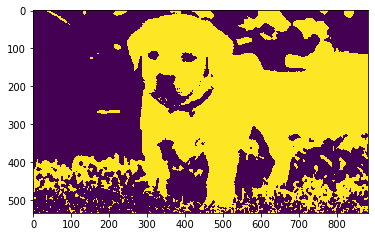Open In App

# Mahotas – Eroding Image

In this article we will see how we can erode the image in mahotas. Erosion (usually represented by ?) is one of two fundamental operations (the other being dilation) in morphological image processing from which all other morphological operations are based. It was originally defined for binary images, later being extended to grayscale images, and subsequently to complete lattices.

In this tutorial we will use “luispedro” image, below is the command to load it.

`mahotas.demos.load('luispedro')`

Below is the luispedro image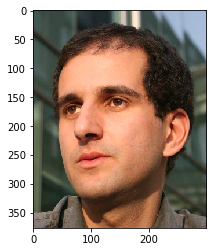In order to do this we will use mahotas.morph.erodemethod

Syntax : mahotas.morph.erode(image)
Argument :It takes image object as argument
Return : It returns image object

Note : Input image should be filtered or should be loaded as grey

In order to filter the image we will take the image object which is numpy.ndarray and filter it with the help of indexing, below is the command to do this

`image = image[:, :, 0]`

Below is the implementation

## Python3

 `# importing required libraries``import` `mahotas``import` `mahotas.demos``from` `pylab ``import` `gray, imshow, show``import` `numpy as np` `# loading image``luispedro ``=` `mahotas.demos.load(``'luispedro'``)` `# filtering image``luispedro ``=` `luispedro.``max``(``2``)` `# otsu method``T_otsu ``=` `mahotas.otsu(luispedro)`` `  `# image values should be greater than otsu value``img ``=` `luispedro > T_otsu` `print``(``"Image threshold using Otsu Method"``)` `# showing image``imshow(img)``show()` `# eroding image``new_img ``=` `mahotas.morph.erode(img)` `# showing eroded image``print``(``"Eroded Image"``)``imshow(new_img)``show()`

Output :

`Image threshold using Otsu Method `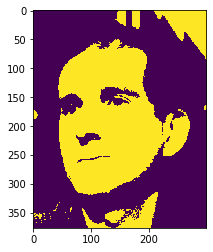`Eroded Image `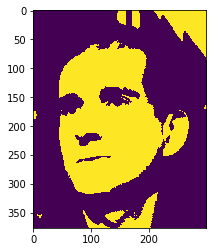Another example

## Python3

 `# importing required libraries``import` `mahotas``import` `numpy as np``import` `matplotlib.pyplot as plt``import` `os`` ` `# loading image``img ``=` `mahotas.imread(``'dog_image.png'``)``      ` `# setting filter to the image``img ``=` `img[:, :, ``0``]` `# otsu method``T_otsu ``=` `mahotas.otsu(img)`` `  `# image values should be greater than otsu value``img ``=` `img > T_otsu` `print``(``"Image threshold using Otsu Method"``)` `# showing image``imshow(img)``show()` `# eroding image``new_img ``=` `mahotas.morph.erode(img)` `# showing eroded image``print``(``"Eroded Image"``)``imshow(new_img)``show()`

Output :

`Image threshold using Otsu Method `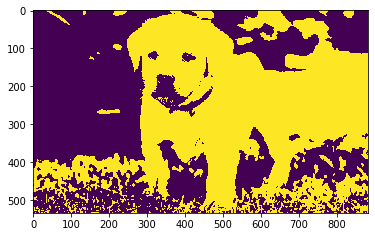`Eroded Image`